02 Apr
02Apr

Differential Pressure Technologies:

Differential Pressure flow meters are, by far the most common technology in use today. Estimates are that over 50% of all liquid flow measurement applications use this type of technology. Head type differential pressure flow meters generate a differential pressure by means of an orifice plate, venture, flow nozzle, pitot tube or elbow tap just to name a few. The number one consideration when choosing a flow meter is a clear understanding of the requirements of a particular application. All of these factors play an integral part in the accuracy and range of differential pressure flow meters. The media being monitored can influence the materials of construction and service life of the flow meter which are important considerations when choosing the right flow meter.

The basic operating principle of differential pressure flow meters is based on the premise that the pressure drop across the meter is proportional to the square of the flow rate. The flow rate is obtained by measuring the pressure differential and extracting the square root.

Head type flow measurement was developed using Bernoulli’s Law an equation describing fluid flow which states that the sum of the pressure head (static energy), the velocity head (kinetic energy), and elevation head (potential energy) at one point is equal to their sum at the other point. Therefore, the energy of the fluid is conserved by continuity and flow across a constriction in the pipe.

Bernoulli type orifice flow meter is a simple and reliable method of measuring fluid flow rate and velocity. Friction losses in the system are accounted for by calibrating the flow meter with a discharge coefficient Cd. Orifice flow meters can be very accurate if properly calibrated. For high accuracy a length of straight pipe is required upstream from the flow meter to minimize turbulent effects from bends and fittings. The flow rate through the nozzle (orifice) is calculated using Bernoulli's equation from the difference in dynamic pressure.

 Operating Principal - Nozzle Flow MeterDifferential pressure flow meters, like most flow meters have a primary and secondary element. The primary element causes a change in kinetic energy, which creates the differential pressure in the pipe. The unit must be properly matched to the pipe size, flow condition, and the liquid or gas properties. In addition, the measurement accuracy of the element must be good over a reasonable range. The secondary element measures the differential pressure and provides the signal or read-out that is converted to the actual flow rate value. The illustration utilizes a pair of metal bellows to sense the differential pressure across a calculated flow nozzle considering the following variables fluid or gas type, operating pressure, operating temperature, pipe size, viscosity, specific gravity, flow rate and differential pressure used to calculate the restriction. Smooth entry and sharp exit. Permanent pressure loss is on the same level as an orifice plate, with the added ability to handle dirty and abrasive fluids. A differential pressure transmitter is used to measure pressure between the nozzle and the pipe flow stream. This type of differential pressure flow meter technology can be constructed to measure either gas or liquids. With a fixed orifice the inlet conditions are important especially in a gas application. The following formulas are be used to obtain the actual flow rate.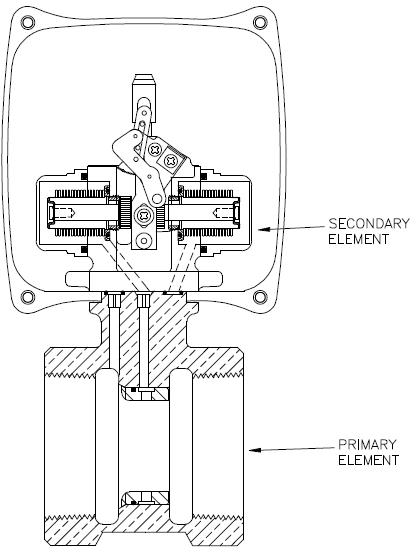Compressed Gas Service

If the gas pressure or temperature varies from the values marked on the flow meter dial, then a correction factor, K, must be applied to the flow meter reading to obtain the actual flow rate.

Actual flow rate = K x flow meter reading

Where:

 K =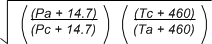Pa = Actual gas pressure at inlet to flow meter, psig

Pc = Calibrated gas pressure marked on the flow meter dial, psig

Ta = Actual gas temperature at inlet to flow meter, °F

Tc = Calibrated gas temperature marked on the flow meter dial, °F

Example:

Flow meter calibrated for compressed gas air at 100°F and 80 psig, reads 100 SCFM at an actual temperature of 70°F and pressure of 85 psig. What is the actual flow rate?

 K =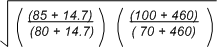K =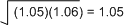Therefore, actual flow rate = 1.05 x 100 = 105 SCFM

Liquid Service

If the specific gravity of the fluid being metered differs from the value marked on the flow meter dial, a correction factor, J, must be applied to the flow meter reading to obtain the actual flow rate.

Actual flow rate = J x flow meter reading

Where: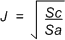Sc = Specific Gravity for which the flow meter is calibrated

Sa = Actual specific gravity of the fluid being metered.

Example:

Flow meter, calibrated for water (specific gravity = 1.0) reads 12.5 GPM for a lubricating oil with a specific gravity of 0.85. What is the actual flow rate?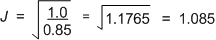Therefore, actual flow = 1.085 x 12.5 = 13.56 GPM

With this type of measurement device, the time it takes for the volume of fluid to pass through the orifice is proportional to the fluid viscosity. Kinematic viscosity is given in units of centistokes while the dynamic viscosity is given in centipoise and the conversion from dynamic to kinematic is given by dividing the dynamic viscosity by the fluid density in g/cm3.

Other differential pressure technologies include orifice plate, venture, pitot tube, elbow tap, nozzle and transducer (pressure sensor). When choosing the right differential pressure flow meter consideration in the corrosive nature, accuracy, cost, conditions and ease of installation must be taken into account for successful monitoring of the process application. Differential pressure flow meters are widely used in a variety of industrial applications such as mining, pulp and paper, petrochemical, mineral processing, water and waste water.

One of the most important developments in DP flowmeters in the past five to ten years has been the packaging of DP transmitters together with primary elements to create a true DP flowmeter. Instead of having to buy and install an orifice plate or averaging pitot tube separately, this new style of DP flowmeter comes with the primary element already integrated with the transmitter. This technology utilizes a pressure sensor that provides a 4-20mA signal to a digital display readout and/or remote location (control room).